# Circle - 9th grade (14y) - math problems

#### Number of problems found: 285

• Circle inscribed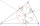There is a triangle ABC and a circle inscribed in this triangle with radius 15. The point T is the point of contact of the inscribed circle with the side BC. What is the area of the triangle ABC if | BT | = 25 a | TC | = 26?
• The spinner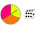The spinner below is spun 12 times. It landed on I 4 times, II 7 times, and III 1 time. What is the difference between the experimental and theoretical probabilities of landing on the II?
• Wheel gearA drive wheel of radius 2 is connected to a drive wheel of radius 1 by a pulley of length 17. What is the distance between the wheel axles?
• Length of the chord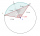Calculate the length of the chord in a circle with a radius of 25 cm with a central angle of 26°.
• The volleyball ball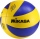The volleyball ball can have a circumference after inflation of at least 650 max 750 mm. What volume of air can this ball hold, if its circumference is the average of the minimum and maximum inflation of the ball.
• Top-open tank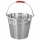The top-open tank has the shape of a truncated rotating cone, which stands on a smaller base. The tank's volume is 465 m3, the radii of the bases are 4 m and 3 m. Find the depth of the tank.
• Woman's day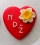We can easily make a heart for mothers for Woman's day by drawing two semicircles to the two upper sides of the square standing on their top. What is the radius of the circle circumscribed by this heart when the length of the side of the square is 1?
• MegapizzaMegapizza will be divided among 100 people. First gets 1%, 2nd 2% of the remainder, 3rd 3% of the remainder, etc. Last 100th 100% of the remainder. Which person got the biggest portion?
• Metal washersMetal washers with a diameter of 80 mm are cut from a strip of steel sheet with a width of 10 cm and a length of 2 m. Calculate the percentage of material waste if no material is lost when two adjacent circles meet.
• The amphitheater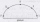The amphitheater has the shape of a semicircle, the spectators sit on the perimeter of the semicircle, the stage forms the diameter of the semicircle. Which of the spectators P, Q, R, S, T sees the stage at the greatest viewing angle?
• The resistanceWhat is the resistance of an aluminum wire 0.2 km long and 10 mm in diameter?
• Rotary motionFind the orbital time, rotational frequency, and angular rate of the second and minute hands on the watch.
• Right triangleA circle with a radius of 5 cm is described in a right triangle with a 6 cm leg. What is the height at the hypotenuse of this triangle?
• Constant Angular Acceleration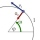The particle began to move from rest along a circle with a constant angular acceleration. After five cycles (n = 5), its angular velocity reached the value ω = 12 rad/s. Calculate the magnitude of the angular acceleration ε of this motion and the time int
• From plasticine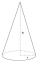Michael modeled from plasticine a 15 cm high pyramid with a rectangular base with the sides of the base a = 12 cm and b = 8 cm. From this pyramid, Janka modeled a rotating cone with a base diameter d = 10 cm. How tall was Janka's cone?
• Two vases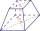Michaela has two vases in her collection. The first vase has the shape of a cone with a base diameter d = 20 cm; the second vase has the shape of a truncated cone with the lower base d1 = 25 cm and with the diameter of the upper base d2 = 15 cm. Which vas
• Five circlesOn the line segment CD = 6 there are 5 circles with a radius one at regular intervals. Find the lengths of the lines AD, AF, AG, BD, and CE.
• 9-gon pyramidCalculate the volume and the surface of a nine-sided pyramid, the base of which can be inscribed with a circle with radius ρ = 7.2 cm and whose side edge s = 10.9 cm.
• Surface and volumeFind the surface and volume of the rotating cone if the circumference of its base is 62.8 m and the side is 25 m long.
• Sphere parts, segmentA sphere with a diameter of 20.6 cm, the cut is a circle with a diameter of 16.2 cm. .What are the volume of the segment and the surface of the segment?

Do you have an exciting math question or word problem that you can't solve? Ask a question or post a math problem, and we can try to solve it.

We will send a solution to your e-mail address. Solved examples are also published here. Please enter the e-mail correctly and check whether you don't have a full mailbox.

Circle Problems. Examples for 9th grade.